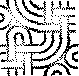Differences between revisions 6 and 7
 ⇤ ← Revision 6 as of 2013-12-27 05:23:36 → Size: 381 Editor: host32 Comment: ← Revision 7 as of 2013-12-27 21:52:00 → ⇥ Size: 3908 Editor: JunHu Comment: Deletions are marked like this. Additions are marked like this. Line 1: Line 1: Guillermo Chumley is what my wife loves to get in touch with me but I never ever actually favored that title. Tennessee has often been my property. Watching motion pictures is what love undertaking. Supervising is exactly where my principal cash flow arrives from and it is some thing I truly enjoy.<
><
>my web site; pure acai ([[http://li-nk.us/3BP|http://li-nk.us]]) #language enPlease feel free to experiment here, after the four dashes below... and please do '''NOT''' create new pages without any meaningful content just to try it out!'''Tip:''' Shift-click "HelpOnEditing" to open a second window with the help pages.----== Formatting ==''italic'' '''bold''' {{{typewriter}}} backtick typewriter (configurable)~+ bigger +~ ~- smaller -~{{{preformatted some moreand some more lines too}}}{{{#!pythondef syntax(highlight):    print "on"    return None}}}== Linking ==HelpOnEditing MoinMoin:InterWiki http://moinmoin.wikiwikiweb.de/ [[http://www.python.org/|Python]]someone@the.inter.net=== Image Link ==={{http://c2.com/sig/wiki.gif}}== Smileys ==/!\ Alert== Lists ===== Bullet === * first   1. nested and numbered   1. numbered lists are renumbered * second * third   mal sehen blockquote   deeper=== Glossary === Term:: Definition=== Drawing ==={{drawing:mytest}}= Heading 1 === Heading 2 ===== Heading 3 ======= Heading 4 ========= besonders gross =====ПростоТест= IRC Log test ={{{#!irc(23:18) < jroes> ah(23:19) < jroes> hm, i like the way {{{ works, but i was hoping the lines would wrap(23:21) -!- gpciceri [~gpciceri@host181-130.pool8248.interbusiness.it] has quit [Read error: 110 (Connection timed out)](23:36) < ThomasWal> you could also write a parser or processor(23:38) < jroes> i could?(23:38) < jroes> would that require modification on the moin end though?(23:38) < jroes> i cant change the wiki myself :x(23:39) < ThomasWal> parsers and processors are plugable(23:39) < ThomasWal> so you dont need to change the core code(23:40) < ThomasWal> you need to copy it to the wiki data directory though(23:40) < jroes> well, what i meant to say was that i dont have access to the box running the wiki(23:40) < ThomasWal> then this is no option awdsd asdasd sa asdasd sad asdadasds adasd asd asd asd asd asd a dadad ad adad ad asd asd adad asdasd asd adad as d(23:40) < jroes> yeah :/}}}[[新ページ]]{{{#!dotdigraph G {  node [fillcolor=white, style=filled];  0 [label="And"];  0 -> 1;  1 [label="a", shape=box];  0 -> 2;  2 [label="Or"];  2 -> 1;  2 -> 3;  3 [label="b", shape=box];  0 -> 4;  4 [label="Or"];  4 -> 3;  4 -> 5;  5 [label="c", shape=box];}}}standard formula:If f is entire (holomorphic on [[latex2(\usepackage{dsfont} % $$\mathds{C}$$)]]) and without zeroes, for every closed curve [[latex2($$\gamma$$)]] the integral [[latex2($$\oint_\gamma \frac{f'(z)}{f(z)}dz$$)]] is zero.This is a red square:{{{#!latex2\usepackage{graphics,color}%%end-prologue%%\newsavebox{\mysquare}\savebox{\mysquare}{\textcolor{red}{\rule{1in}{1in} } }\usebox{\mysquare}}}} This is a Z notation:{{{#!latex2\usepackage{oz}\setlength{\zedcornerheight}{0.2em}%%end-prologue%%\small\begin{schema}{level2\_robot}    \textbf{in~} x: \mathbb{B} \\    \textbf{in~} feeling: \mathbb{R} \\    \textbf{in~} control: \mathbb{C} \\\zbreak    \textbf{out~} moving: \mathbb{R} \\    \textbf{out~} u: \mathbb{B} \\\ST\zbreak    control.1.1 = x2moving \imp \\        \t1 maxrate(x, $10K$) \\        \t1 \land delay(pr''(x \copyright DBH), moving, \Delta_{level2\_robot}) \\\zbreak    control.1.1 = feeling2u \imp \\        \t1 maxrate(u, $10K$) \\        \t1 \land delay(feeling, pr''(u \copyright IBH), \Delta_{level2\_robot}) \\\zbreak    control.1.1 = x2moving\_feeling2u \imp \\        \t1 maxrate(x, $10K$) \\        \t1 \land maxrate(u, $10K$) \\        \t1 \land delay(pr''(x \copyright DBH), moving, \Delta_{level2\_robot}) \\        \t1 \land delay(feeling, pr''(u \copyright IBH), \Delta_{level2\_robot}) \\\end{schema}}}} ----

Please feel free to experiment here, after the four dashes below... and please do NOT create new pages without any meaningful content just to try it out!

Tip: Shift-click "HelpOnEditing" to open a second window with the help pages.

## Formatting

italic bold typewriter

backtick typewriter (configurable)

bigger smaller

preformatted some more
and some more lines too

   1 def syntax(highlight):
2     print "on"
3     return None## SmileysAlert

## Lists

### Bullet

• first
1. nested and numbered
2. numbered lists are renumbered
• second
• third
• mal sehen
blockquote
• deeper

Term
Definition

# IRC Log test

   1 (23:18) <     jroes> ah
2 (23:19) <     jroes> hm, i like the way {{{ works, but i was hoping the lines would wrap
3 (23:21) -!- gpciceri [~gpciceri@host181-130.pool8248.interbusiness.it] has quit [Read error: 110 (Connection timed out)]
4 (23:36) < ThomasWal> you could also write a parser or processor
5 (23:38) <     jroes> i could?
6 (23:38) <     jroes> would that require modification on the moin end though?
7 (23:38) <     jroes> i cant change the wiki myself :x
8 (23:39) < ThomasWal> parsers and processors are plugable
9 (23:39) < ThomasWal> so you dont need to change the core code
10 (23:40) < ThomasWal> you need to copy it to the wiki data directory though
11 (23:40) <     jroes> well, what i meant to say was that i dont have access to the box running the wiki
13 (23:40) <     jroes> yeah :/


digraph G {
node [fillcolor=white, style=filled];
0 [label="And"];
0 -> 1;
1 [label="a", shape=box];
0 -> 2;
2 [label="Or"];
2 -> 1;
2 -> 3;
3 [label="b", shape=box];
0 -> 4;
4 [label="Or"];
4 -> 3;
4 -> 5;
5 [label="c", shape=box];

standard formula: If f is entire (holomorphic on latex2(\usepackage{dsfont} % $$\mathds{C}$$)) and without zeroes, for every closed curve latex2($$\gamma$$) the integral latex2($$\oint_\gamma \frac{f'(z)}{f(z)}dz$$) is zero.

This is a red square:

\usepackage{graphics,color}
%%end-prologue%%
\newsavebox{\mysquare}
\savebox{\mysquare}{\textcolor{red}{\rule{1in}{1in} } }
\usebox{\mysquare}

This is a Z notation:

\usepackage{oz}
\setlength{\zedcornerheight}{0.2em}
%%end-prologue%%
\small
\begin{schema}{level2\_robot}
\textbf{in~} x: \mathbb{B} \\
\textbf{in~} feeling: \mathbb{R} \\
\textbf{in~} control: \mathbb{C} \\
\zbreak
\textbf{out~} moving: \mathbb{R} \\
\textbf{out~} u: \mathbb{B} \\
\ST
\zbreak
control.1.1 = x2moving \imp \\
\t1 maxrate(x, $10K$) \\
\t1 \land delay(pr''(x \copyright DBH), moving, \Delta_{level2\_robot}) \\
\zbreak
control.1.1 = feeling2u \imp \\
\t1 maxrate(u, $10K$) \\
\t1 \land delay(feeling, pr''(u \copyright IBH), \Delta_{level2\_robot}) \\
\zbreak
control.1.1 = x2moving\_feeling2u \imp \\
\t1 maxrate(x, $10K$) \\
\t1 \land maxrate(u, $10K$) \\
\t1 \land delay(pr''(x \copyright DBH), moving, \Delta_{level2\_robot}) \\
\t1 \land delay(feeling, pr''(u \copyright IBH), \Delta_{level2\_robot}) \\
\end{schema}

JunHu: WikiSandBox (last edited 2013-12-27 21:52:00 by JunHu)